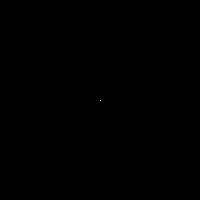# How to use Pillow (PIL: Python Imaging Library)

Posted: 2019-05-14 / Tags: Python, Pillow, Image Processing

Pillow is an image processing library that has been forked from PIL (Python Image Library) that development has been stopped.

Pillow is the “friendly PIL fork” by Alex Clark and Contributors. PIL is the Python Imaging Library by Fredrik Lundh and Contributors. Pillow — Pillow (PIL Fork) 4.2.1 documentation

Although advanced image processing (face recognition, optical flow, etc.) like OpenCV can not be performed, simple image processing such as resizing (scaling), rotation, and trimming (partial cutout) can be performed.

Because Pillow is simpler and easier to understand than OpenCV, it is better to use it depending on the purpose.

• Install
• Basic usage
• Drawing
• Other processing example

See the following link for individual articles that explain various processes such as resizing and trimming.

## Install

Pillow can be installed by `pip`.

``````\$ pip install Pillow
``````

Pillow and PIL can not coexist. If you have installed both, you should uninstall both and reinstall only one.

If you do not know what is installed, you can check it as follows.

``````\$ pip list
``````

Uninstall is performed as follows.

``````\$ pip uninstall <package>
``````

Note that if you are using Python 3, you may have to use `pip3` instead of `pip` depending on your environment.

## Basic usage

The official document is easy to understand.

Read image file. Note that the name of the package to import is `PIL`, not `Pillow`.

``````from PIL import Image, ImageFilter

im = Image.open('data/src/lenna_square.png')
``````

Get meta information such as format, size (width, height), mode, etc. The size is expressed by `(width, height)`.

``````print(im.format, im.size, im.mode)
# PNG (512, 512) RGB
``````

Get minimum value and maximum value of each color of RGB.

``````print(im.getextrema())
# ((54, 255), (3, 248), (8, 225))
``````

Get the pixel value of the specified coordinate. The coordinate origin `(0, 0)` is upper left.

``````print(im.getpixel((256, 256)))
# (180, 65, 72)
``````

As an example, processing of gray scale conversion (`convert('L')`), rotation by 90 degrees (`rotate(90)`), and Gaussian blur (`filter()`) is performed.

``````new_im = im.convert('L').rotate(90).filter(ImageFilter.GaussianBlur())
``````

Display images with OS default software.

``````new_im.show()
``````

Save the image.

``````new_im.save('data/dst/lenna_square_pillow.jpg', quality=95)
``````

When saving with `save()`, the parameters differ depending on the extension. See Image file format for details. In the case of jpg, you can specify the quality with the parameter `quality`. The default is 75 for 1 (lowest) to 95 (highest).

The original image and the image after processing are as follows.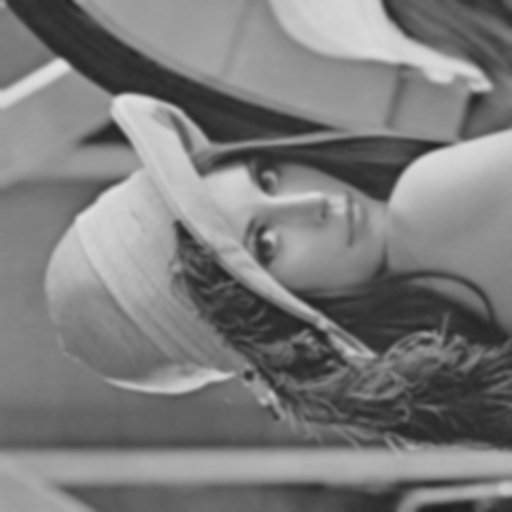### Drawing

You can also draw figures.

First, create a solid image. Specify the size in the second argument and RGB colors in the third argument.

``````from PIL import Image, ImageDraw, ImageFont

im = Image.new("RGB", (512, 512), (128, 128, 128))
``````

Specify the `Image` object as an argument and create a `Draw` object.

``````draw = ImageDraw.Draw(im)
``````

Draw straight line, rectangle and ellipse.

``````draw.line((0, im.height, im.width, 0), fill=(255, 0, 0), width=8)
draw.rectangle((100, 100, 200, 200), fill=(0, 255, 0))
draw.ellipse((250, 300, 450, 400), fill=(0, 0, 255))
``````

Draw text with specified font.

``````font = ImageFont.truetype('/Library/Fonts/Arial Bold.ttf', 48)
draw.multiline_text((0, 0), 'Pillow sample', fill=(0, 0, 0), font=font)
``````

The result is as follow.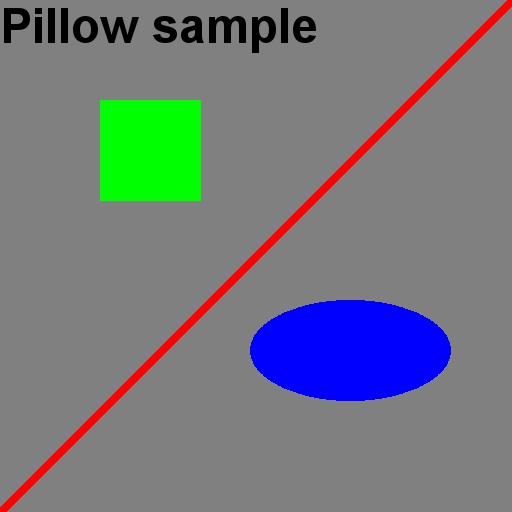In this example, a solid image is generated first, but it is also possible to read an image file and draw figures and text on it.

## Other processing example

Here are some examples of image processing using Pillow (PIL). For details and sample code, refer to the articles linked.

### Paste another image into the image

Paste another image on the background image.

In addition to rectangles, it is also possible to cut out and paste in any shape such as a circle using a mask image.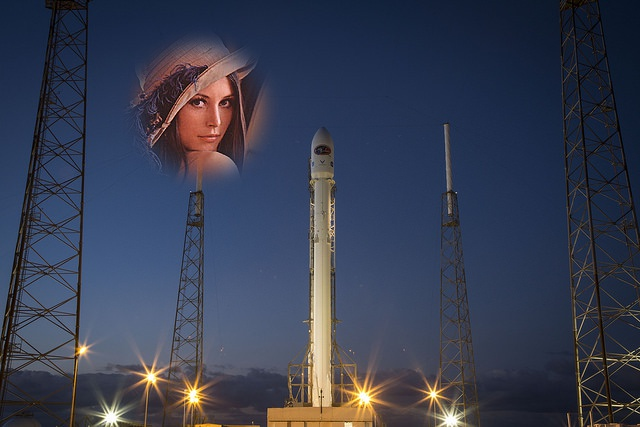### Composite two images

Composite two images of the same size.

You can compose according to a mask image. A gradation image can be used as a mask to create an image that changes gradually.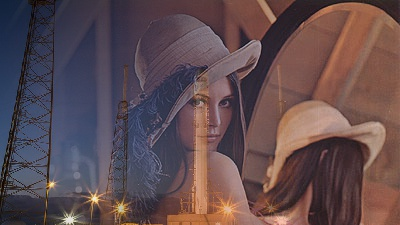### Concatenate multiple images

Multiple images can be arranged vertically or horizontally to create a concatenated image.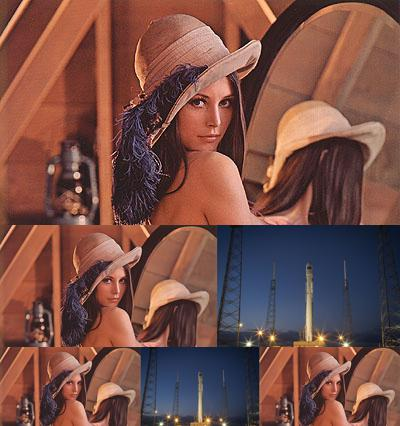### Create transparent png image

Create a transparent png image with an alpha channel.

This too can be masked in any shape.### Negative / positive inversion (invert pixel value)

A negative-positive inverted image (image with inverted pixel values) is generated.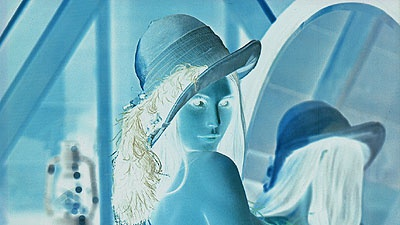### Create circular or square thumbnail images

Create thumbnail images from large images.

You can cut out the center, add margins to make it any size, or make it circular. It is also possible to process multiple images at once.### Create animated gif

You can create animated gif image as well as jpg and png.